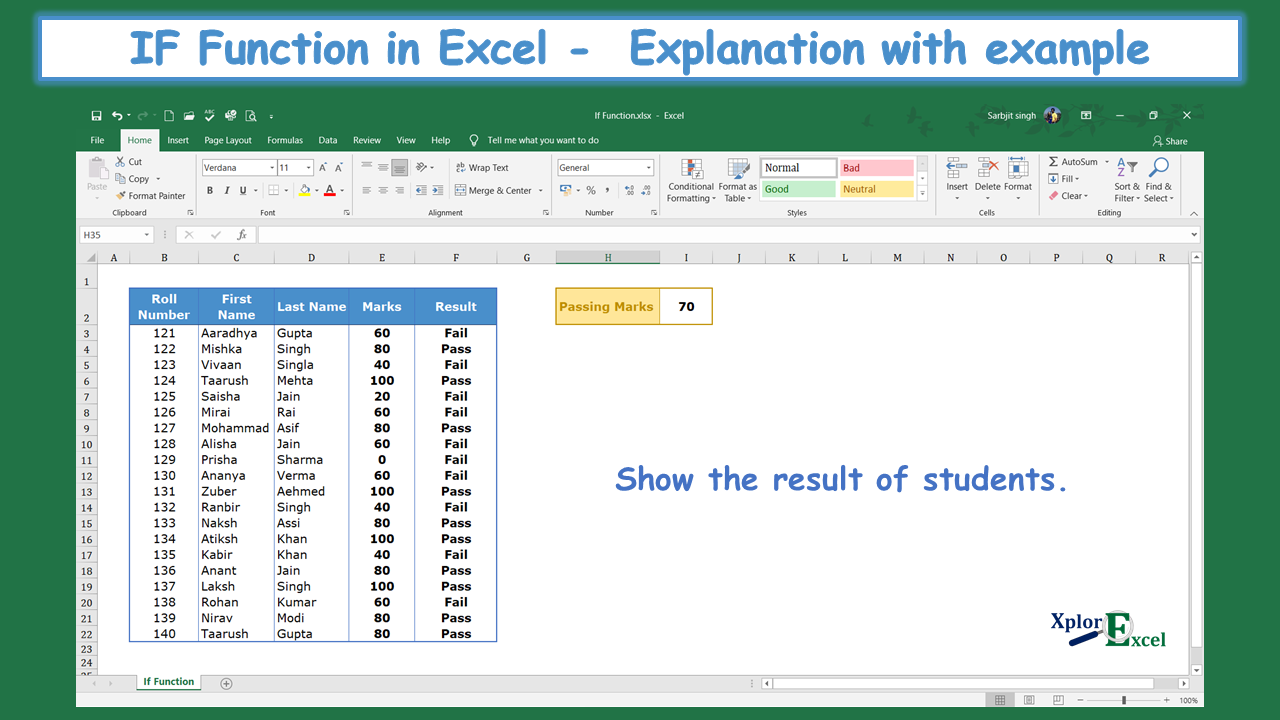## IF Function in Excel: –

The “IF” Function in Excel is a logical test, representing the result in two ways if the logical condition is true and false. There is always two way of getting the value, one is when we got our condition/logic true and another we will get when the condition/logic false. We can use this formula where we want to apply the condition of less than, greater than, and equal to. It means if the selected cell’s value is less than a specific amount, then answer the specific true value(it may be in numeric or in words) rather than answer the false value.

### The feature of the “IF” function: –

• With the help of the IF Function, we can retrieve the data if the condition is cleared by the data.
• VLOOKUP and HLOOKUP shows only matched value but the “IF” function shows the value in both ways.
• It helps in applying a particular condition to the data.

### The logical operator: –

We can use the “IF” function only to follow a logical test on the selected array.

 Logical Test Meaning = Equal to <> Not Equal to > Greater than >= Greater than and Equal to < Less than <= Less than and equal to

### Explanation of Function:-

Now, We will explain the Arguments of the Function.

=IF(logical_test, [value_if_true], [value_if_false])

logical_test:- The Logical test is the value or condition which will provide us with the result of the selected cell as true or false. It is a specific condition/test as explained in the above table.

value_if_true: – The value if true is that value which you want to show if the condition or logical test is true.

value_if_false: – The value if false is that value which you want to show if the condition or logical test is false.

### Example of Function: –

In the following table, we want to retrieve the result of all students in the Pass and Fail Form. The minimum passing marks are 70.Solution:-

We will Apply the if function and get the result. This is shown in the following steps: –

Step No. 1:-

We will write the “=if( ” in the column of the result.

Step No. 2

Now select the cell on which you want to apply the logical test. and then write the logical test

Or if can by selecting the cell where the value of logic is written and then fix this cell by adding “\$” signs.

Step No. 3: –

Now, write the answer which you want if the condition show when the condition is true. Write the answer in t invited comma.Example of If – Solution Step no. 3

Step No. 4: –

Now, write the answer which you want if the condition show when the condition is false. Write the answer in t invited comma.

Step No. 5: –

Now, Copy and paste the function in all remaining cells to get the result.

Thanks

Also, Check out the following function of excel: –

#### Social Share### Sarbjit Singh

1.#### taranjot

January 30, 2021

cant understand from step 2, why use E3, why \$ sign

1.#### Sarbjit

January 30, 2021

E3 cell is highlighted with the light blue color.
and ‘\$’ is used to fix the cell. when you copy this formula in any other cell then the selection remains the same for the lookup value.

2. #### Excel SUMIF Function - Download practice Sheet - Xplore Excel

January 30, 2021

[…] IF Function in Excel – Free Explanation with example […]

3. #### Excel Match Function- Download 2 practice Sheet - Xplore Excel

January 30, 2021

[…] IF Function in Excel – Free Explanation with example […]

4. #### COUNT Function in Excel- Download 2 Practice Sheet - Xplore Excel

January 30, 2021

[…] IF Function in Excel – Free Explanation with example […]

5. #### COUNTIF Excel Function - Download 2 Practice Sheets - Xplore Excel

January 30, 2021

[…] IF Function in Excel – Free Explanation with example […]

6. #### Sum Function in Excel - Download 2 Practice Sheets - Xplore Excel

January 30, 2021

[…] IF Function in Excel – Free Explanation with example […]

7.#### zortilo nrel

January 30, 2021

What¦s Going down i’m new to this, I stumbled upon this I’ve discovered It positively useful and it has helped me out loads. I’m hoping to contribute & assist other users like its helped me. Great job.

8.#### zovrelioptor

January 30, 2021

Good info. Lucky me I reach on your website by accident, I bookmarked it.

9.#### zortilonrel

January 30, 2021

Enjoyed reading this, very good stuff, appreciate it.